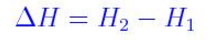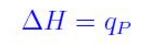# Enthalpy Formula

The variations together with the heat content of the materials which react, result in enthalpy(H) the accurate word for heat content. Typically, this variation in the heat content or enthalpy is displayed by an alteration in temperature. There is an alteration in the heat of the reaction, during the reaction. The enthalpy change for the reaction is represented by  ΔH (sometimes called the heat of reaction). The enthalpy of products is H2 and is less than the heat content of reactants H1.

Enthalpy Formula is denoted as

Enthalpy Change = Heat of the ReactionAs the enthalpy change amplifies itself as heat, the statement “heat of reaction” is frequently made use of in place of enthalpy change of the reaction. The key relation between enthalpy change and heat of reaction. The enthalpy of reaction equals the heat of reaction at constant pressure.

## Solved Examples

Problem 1: For the thermite reaction compute the enthalpies of formation.

2Al + Fe2O3 àAl2O3 + 2Fe

This reaction occurs when a mixture of powders aluminium and iron(III) oxide is ignited with a magnesium fuse.

Given

ΔH for Fe2O3 = -826kJ/mol

ΔH for Al2O3 = -1676 kJ/mol

ΔH for Al =  ΔH for Fe = 0

Solution:

We use the equation.

Enthalpy Formula

ΔH (reaction) =  ΔH for Al2O3 –  ΔH for Fe2O3

ΔH (reaction) = -1676kJ – (-826kJ)

ΔH (reaction) = -850kj

Problem 2: For a combustion reaction.

2C8H18 + 25CO2 à 16CO2 + 18H2O

What is the variation in enthalpy of the reaction?

Given

ΔH for CO2 = -394kJ

ΔH for H2O = -286kJ

ΔH for C8H18 = -269kJ

Solution:

ΔH = 16 times  ΔH for CO2 + 18 times  ΔH for H2O – 2 times  ΔH for C8H18

ΔH = 16 times (-394kJ) + 18 times (-286kJ) – 2 times (-269kJ)

ΔH = -1.09 times 104kJ